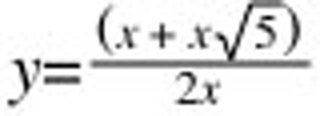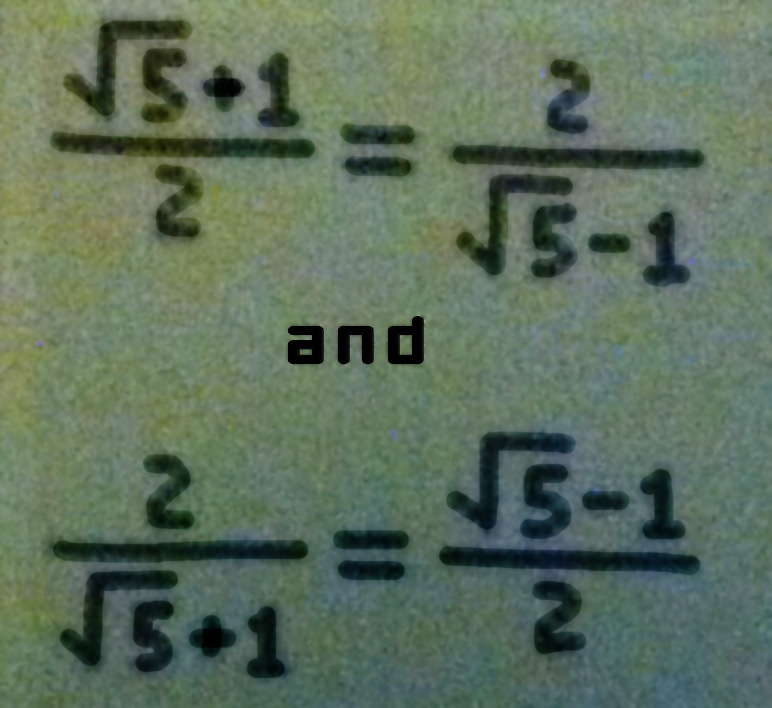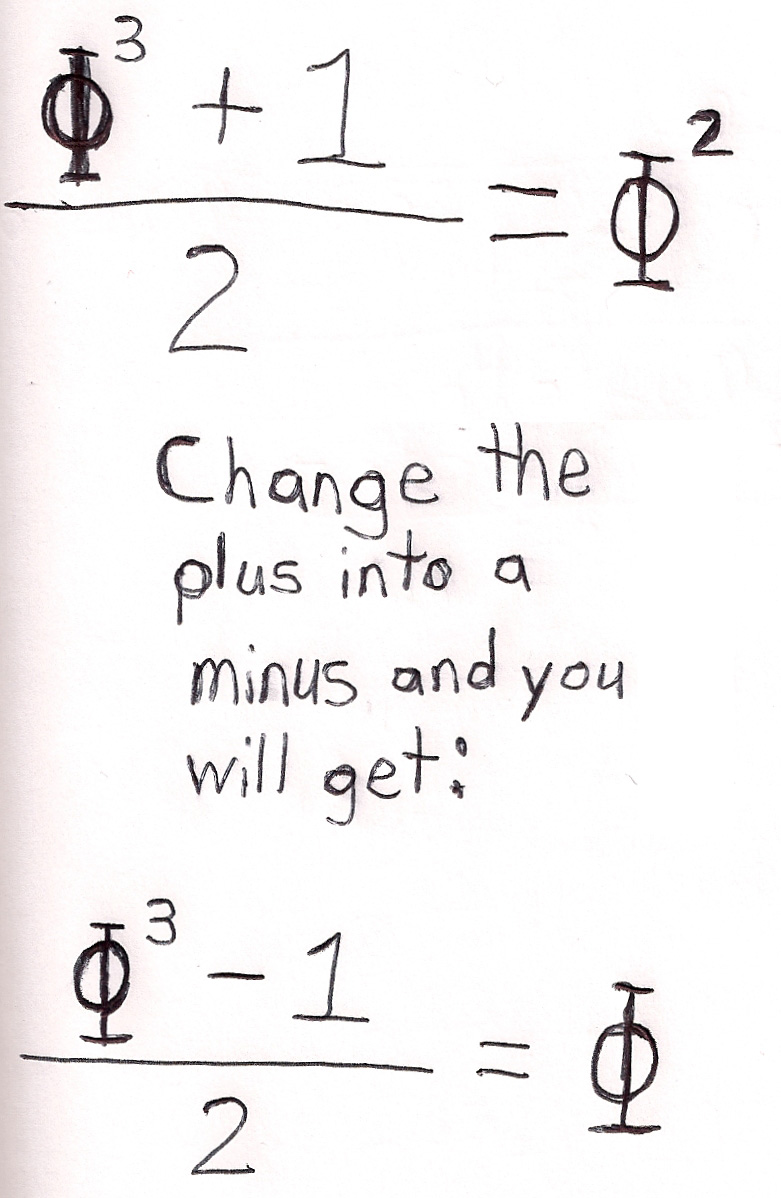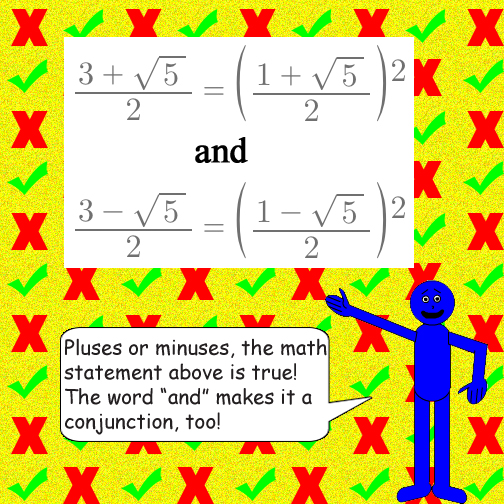# The Irrational Number Phi

## Y = X + 1

### Actually, these functions intersect twice; but at each intersection, X = -φ + 1 or φ. Here's how Phi looks as an algebraic fraction:Note: You can multiply all 3 numbers in the fraction individually by a non-zero number(the exact same multiplier for each of the 3 numbers) to get the exact same ratio!In this function, y will always be equal to φ as long as x ≠ 0!

Also:P.S.: If the numbers φ3 & 1 swap places with the minus sign in-between them, then you'll get because subtracting bigger numbers from smaller numbers makes the difference negative! (φ3 > 1)